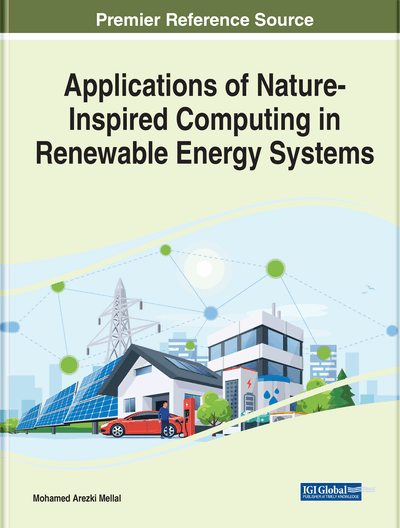# Ant Colony Optimization Algorithm for Electrical Power Systems Applications: A Literature Review

Ragab A. El-Sehiemy (Kafrelsheikh University, Egypt) and Almoataz Y. Abdelaziz (Ain Shams University, Egypt)
DOI: 10.4018/978-1-7998-8561-0.ch004
Available
\$37.50
No Current Special Offers

## Abstract

Optimization has been an active area of research for several decades. As many real-world optimization problems become increasingly complex, better optimization algorithms are always needed. Recently, meta-heuristic global optimization algorithms have become a popular choice for solving complex and intricate problems, which are otherwise difficult to solve by traditional methods. This chapter reviews the recent applications of ant colony optimization (ACO) algorithm in the field of electrical power systems. Also, the progress of the ACO algorithm and its recent developments are discussed. This chapter covers the aspects like (1) basics of ACO algorithm, (2) progress of ACO algorithm, (3) classification of electrical power system applications, and (4) future of ACO for modern power systems application.
Chapter Preview
Top

## 1. Basics Of Ant Colony Optimizer

At first, the ACO algorithms were proposed in ‎(Dorigo & Gambardella, 1997a; Dorigo & Gambardella, 1997b). Applications of the ACO to combinatorial optimization problems such as traveling salesman problem (TSP) (Jun-man & Yi, 2012) and quadratic assignment problem (QAP). The ACO algorithms emulate the behavior of real ants that are members of a family of social insects (Dorigo, 2008; Dorigo & Blum, 2005). A literature survey on the concept and the formulations of ACO was presented in (Dorigo et al., 1999; Dorigo & Stützle, 2009). Ant algorithms for discrete optimization problem were presented (Dorigo et al., 1999; Dréo & Siarry, 2002; Mathur et al., 2000) and for continuous function optimization were developed in (Dréo & Siarry, 2002; Gomez et al., 2004; Mathur et al., 2000). The mathematical model of ACO algorithm involve random number of pheromones that are is placed in each pass after each ant finalizes its tour. Other ants attract to the shortest route according to the probabilistic transition rule that depends on the amount of pheromone deposited and a heuristic guide function. Therefore, the probabilistic transition rule of ant k to go from city i to city j can be expressed as in TSP (Dorigo & Gambardella, 1997a; Dorigo & Gambardella, 1997b; Jun-man & Yi, 2012) as:

(1)

After completing each tour, the local pheromone update is resolute for each ant depending on the route of each ant as in equation (2).

𝜏ij(t + 1) = (1 – 𝜌)𝜏ij(t) + 𝜌𝜏o(2)

After all ants attracted to the shortest route, a global pheromone update is considered to show the influence of the new addition deposits by the other ants that attractive to the best tour as:

𝜏ij(t + 1) = (1 – 𝜌)𝜏ij(t) + 𝜀∆𝜏ij(t)(3)

Then, ∆τij is the amount of pheromone for elite path as:

∆𝜏ij(t) = 𝜆/dbest(4)Top

## 2. Family Of Aco Algorithms

In the literature, there are many variants of the ACO algorithm. Samples of these variants which aim at improving the performance of the basic version. Hybridizations are the combination of the basic algorithm with a local search. The original ant system and the most successful variants are presented. Description of these variants is presented in the following subsections.

### 2.1 Ant System (AS)

The main merits of AS is that the pheromone values are updated at each iteration by all the m ants in the iteration. The associated pheromone 𝜏ij with the edge joining cities i and j is computed from Equation (5) as:

(5)(6) for the solution construction, ants select the following city to be visited through a stochastic mechanism. When ant k is in city i and has so far constructed the partial solution x the probability of going to city j is defined by:

(7)

## Complete Chapter List

Search this Book:
Reset